# 图解堆排序，带你彻底了解清楚！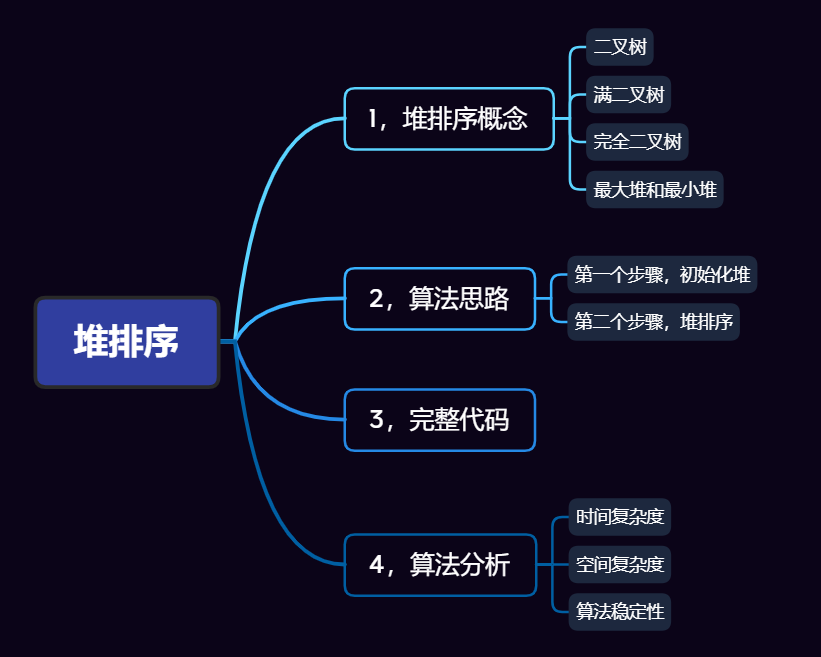## 1，堆排序概念

### 1.1，二叉树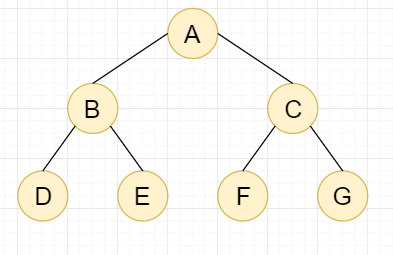### 1.2，满二叉树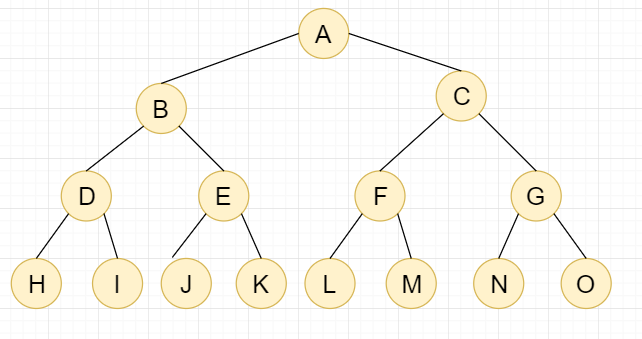### 1.3，完全二叉树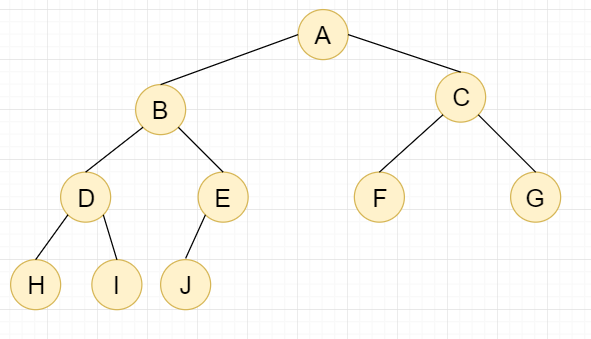## 2，算法思路

int[] arr={4,2,8,0,5,7,1,3,9};

### 2.1，第一个步骤，初始化堆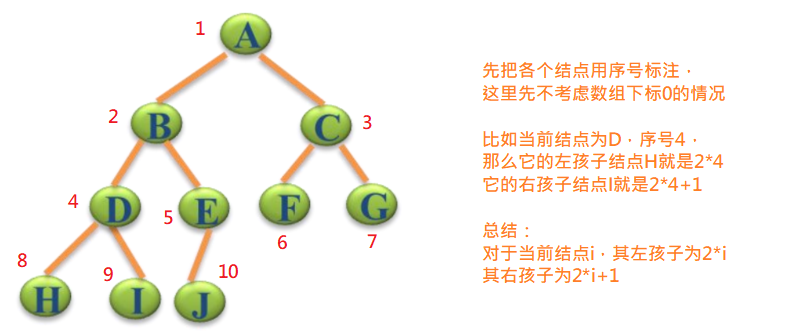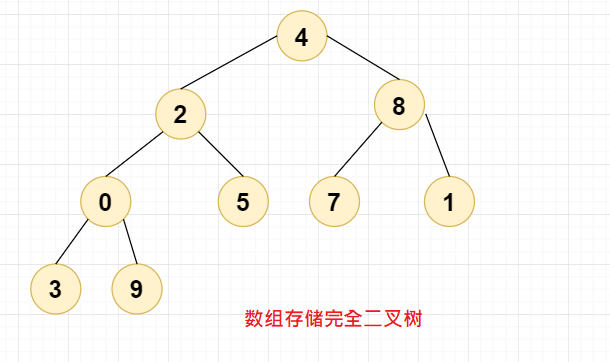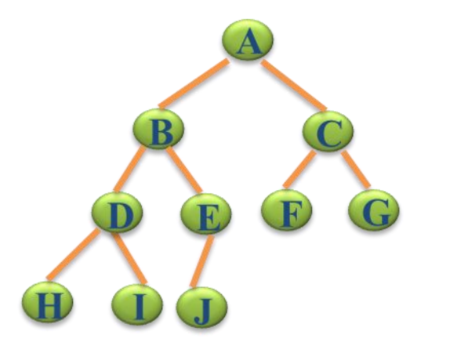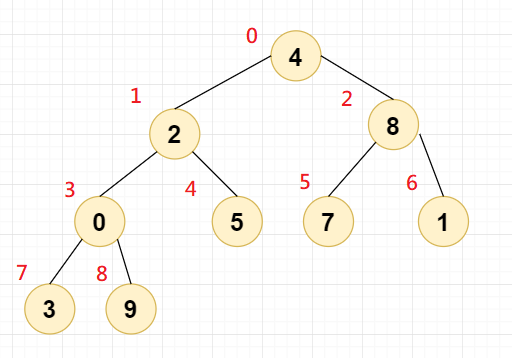//初始化堆public static void HeapAdjust(int[] arr,int index,int len){        //先保存当前节点的下标        int max = index;        //保存左右孩子数组下标        int lchild = index*2+1;        int rchild = index*2+2;        //开始调整，左右孩子下标必须小于len，也就是确保数组不会越界        if(lchild<len && arr[lchild] > arr[max]){            max=lchild;        }        if(rchild<len && arr[rchild] > arr[max]){            max=rchild;        }        //若发生了交换，则max肯定不等于index了。此时max节点值需要与index节点值交换，上面交换索引值，这里交换节点值        if(max!=index){            int temp=arr[index];            arr[index]=arr[max];            arr[max]=temp;            //交换完之后需要再次对max进行调整，因为此时max有可能不满足最大堆            HeapAdjust(arr,max,len);        }    }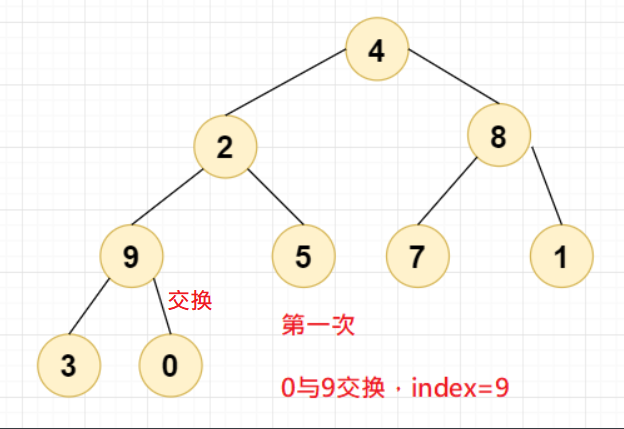0 与 9 交换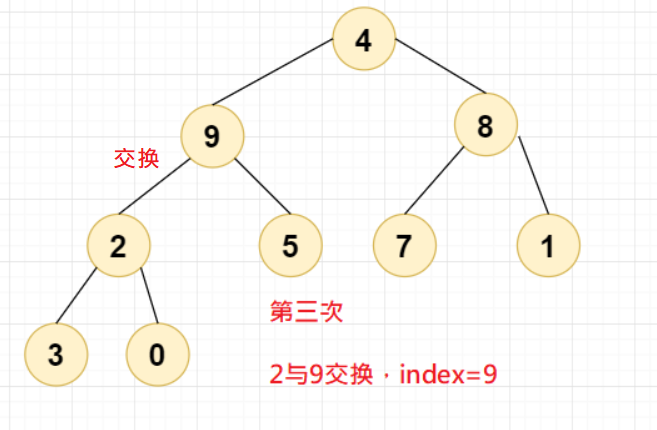2 与 9 交换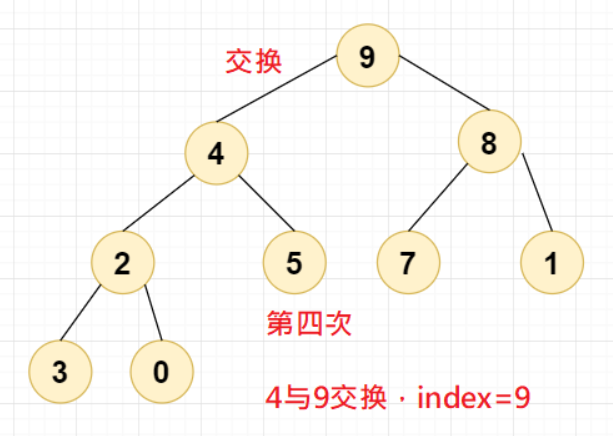4 与 9 交换

//若发生了交换，则max肯定不等于index了。此时max节点值需要与index节点值交换，上面交换索引值，这里交换节点值if(max!=index){    int temp=arr[index];    arr[index]=arr[max];    arr[max]=temp;    //交换完之后需要再次对max进行调整，因为此时max有可能不满足最大堆    HeapAdjust(arr,max,len);}

//堆排序    public static int[] HeapSort(int[] arr){        int len=arr.length;        /**         * 第一步，初始化堆，最大堆，从小到大         * i从完全二叉树的第一个非叶子节点开始，也就是len/2-1开始(数组下标从0开始)         */        for(int i=len/2-1;i>=0;i--){            HeapAdjust(arr,i,len);        }        //打印堆顶元素，应该为最大元素9        System.out.println(arr);        return arr;    }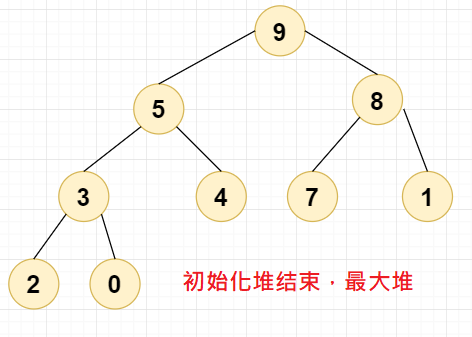### 2.2，第二个步骤，堆排序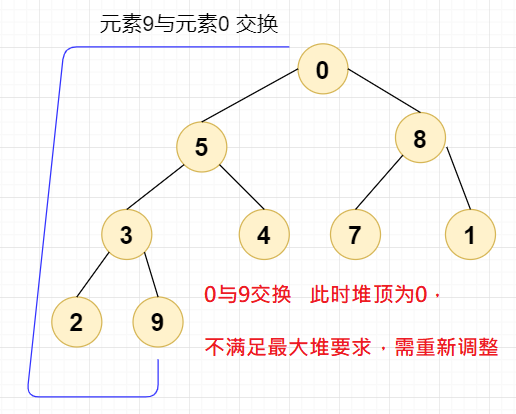0 与 9 交换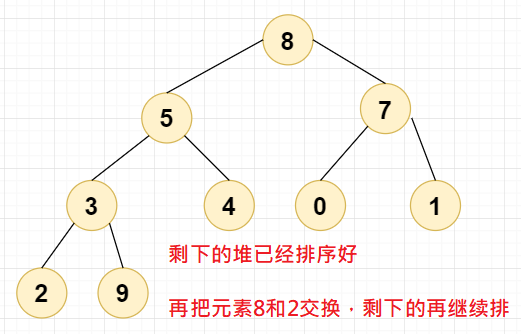/** * 第二步，交换堆顶（最大）元素和二叉堆的最后一个叶子节点元素。目的是交换元素 * i从二叉堆的最后一个叶子节点元素开始，也就是len-1开始(数组下标从0开始) */for(int i=len-1;i>=0;i--){    int temp=arr[i];    arr[i]=arr;    arr=temp;    //交换完之后需要重新调整二叉堆，从头开始调整，此时Index=0    HeapAdjust(arr,0,i);}

HeapAdjust(int[] arr,int index,int len)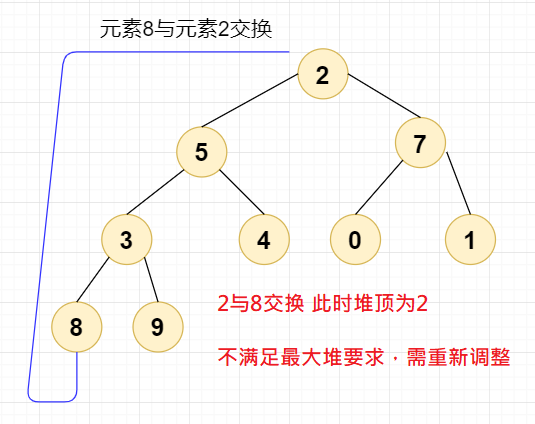8 与 2 交换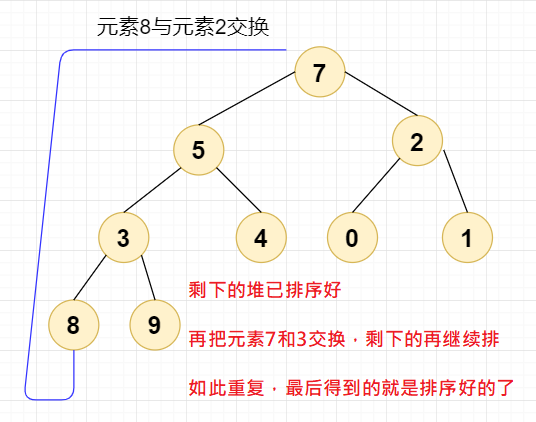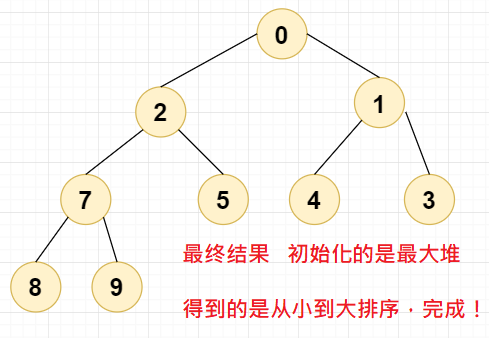## 3，完整代码

import java.util.Arrays;public class Head_Sort {    public static void main(String[] args) {        int[] arr={4,2,8,0,5,7,1,3,9};        System.out.println("排序前:"+Arrays.toString(arr));        System.out.println("排序后:"+Arrays.toString(HeapSort(arr)));    }    //堆排序    public static int[] HeapSort(int[] arr){        int len=arr.length;        /**         * 第一步，初始化堆，最大堆，从小到大。目的是对元素排序         * i从完全二叉树的第一个非叶子节点开始，也就是len/2-1开始(数组下标从0开始)         */        for(int i=len/2-1;i>=0;i--){            HeapAdjust(arr,i,len);        }        /**         * 第二步，交换堆顶（最大）元素和二叉堆的最后一个叶子节点元素。目的是交换元素         * i从二叉堆的最后一个叶子节点元素开始，也就是len-1开始(数组下标从0开始)         */        for(int i=len-1;i>=0;i--){            int temp=arr[i];            arr[i]=arr;            arr=temp;            //交换完之后需要重新调整二叉堆，从头开始调整，此时Index=0            HeapAdjust(arr,0,i);        }        return arr;    }    /**     *初始化堆     * @param arr 待调整的二叉树(数组)     * @param index 待调整的节点下标，二叉树的第一个非叶子节点     * @param len 待调整的数组长度     */    public static void HeapAdjust(int[] arr,int index,int len){        //先保存当前节点的下标        int max = index;        //保存左右孩子数组下标        int lchild = index*2+1;        int rchild = index*2+2;        //开始调整，左右孩子下标必须小于len，也就是确保index必须是非叶子节点        if(lchild<len && arr[lchild] > arr[max]){            max=lchild;        }        if(rchild<len && arr[rchild] > arr[max]){            max=rchild;        }        //若发生了交换，则max肯定不等于index了。此时max节点值需要与index节点值交换，上面交换索引值，这里交换节点值        if(max!=index){            int temp=arr[index];            arr[index]=arr[max];            arr[max]=temp;            //交换完之后需要再次对max进行调整，因为此时max有可能不满足最大堆            HeapAdjust(arr,max,len);        }    }}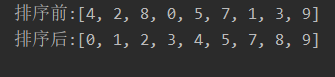## 4，算法分析

### 4.3，算法稳定性## 评论 (1 条评论)21 小时前